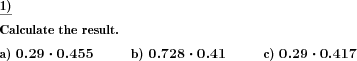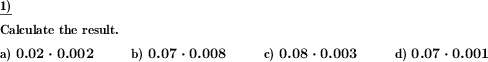Custom math worksheets at your fingertips# Details for problem "Decimal fractions multiplication"

Quickname: 1824

Elementary School, Primary School, Junior High School, Middle School, High School.

## Summary

Two decimals have to be multiplied.

## Examples## Description

Two decimal fractions have to be multiplied.

For the first and second factor, the places before the decimal point and the decimal places can be individually selected.

In addition, the first n decimal places can be made zero digits for each of the two factors. In this way, numbers of different shapes can be generated. As the parameters like number of places, number of decimals and number of leading decimals that are zero, can be chosen for each of the two factors individually, these different number shapes can be combined in a single problem.

Number of places: 0 Number of decimals: 3 Leading decimals=0: 0

```    0.222 * 0,139      0.456 * 0,772      0.999 * 0.111
```

Number of places: 2 Number of decimals: 2 Leading decimals=0: 0

```    12.21 * 34.34      89.12 * 45.23      23.44 * 65.54
```

Number of places: 0 Number of decimals: 3 Leading decimals=0: 2

```    0.002 * 0.003      0.001 * 0.009      0.008 * 0.007
```

The number of problems can be chosen.

Download free printable worksheets for this math problem here. The worksheet contains the problems only, the solution sheet includes the answers. Just click on the respective link.

•Worksheet 1Solution sheet with answers
•Worksheet 2Solution sheet with answers
•Worksheet 3Solution sheet with answers

If you can not see the solution sheets for download, they may be filtered out by an ad blocker that you may have installed. If this is the case, please allow ads for this page and reload the page. The solution sheets will then reappear.

• Do these sample worksheets do not really fit?
• Do you need more math worksheets, with a different level of difficulty?
• Would you like to combine different problems on a worksheet and adjust them to your needs?
• As a teacher, you can put together your own worksheets using the automatically generated math problems provided.
With a free initial credit, you can start creating your own math worksheets in a few minutes.

You can try it for free! Register here, to create custom worksheets now!

## Customization options for this problem

Parameter
Possible values
Number of problems
1, 2, 3, 4, 5, 6, 7, 8, 9, 10
Places 1st number
0, 1, 2, 3
Decimals 1st number
1, 2, 3, 4
1st decimals 0 up to pos
0, 1, 2, 3
Places 2nd number
0, 1, 2, 3
Decimals 2nd number
1, 2, 3, 4
2nd decimals 0 up to pos
0, 1, 2, 3
Swap factors
Yes, No